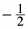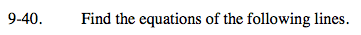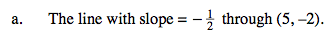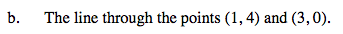### Home > AC > Chapter 16 > Lesson 16.9.1.3 > Problem9-40

9-40.
1. Find the equations of the following lines. Homework Help ✎

1. The line with slope =through (5, − 2).

2. The line through the points (1, 4) and (3, 0).y = mx + b(yy1) = m(xx1)